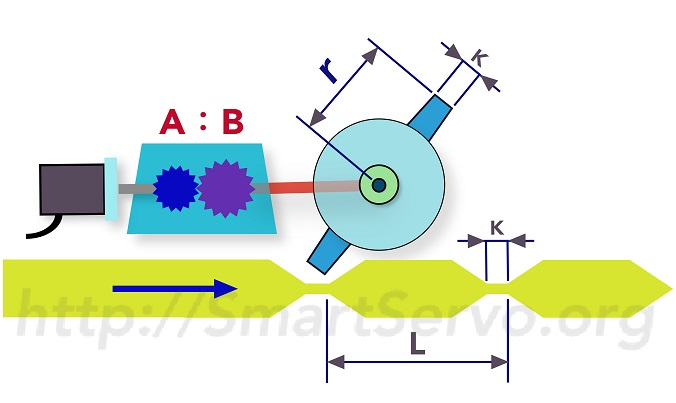# 巨集 #7 飞剪造表（2）公式彙整 与 范例

## 飞剪曲线 公式彙整• 切长比：$R=\frac{L}{\frac{2\pi r}{C}}=\frac{L\cdot C}{2\pi r}$
• 同步区：$Y=\frac{K}{L}\times360^\circ$
• 等待区：$W=?$
•$R<1$，则$W=0$
•$R\geq 1$，则$W=\max(0,w')$，其中$w'=180^\circ+\frac{360^\circ}{P5-82}-\frac{360^\circ}{R}+\frac{Y}{2}$

## 造表范例#1，切长比 R < 1

• 切长比$R=\frac{L\cdot C}{2\pi r}=\frac{160\times 2}{2\pi\times 150}=0.339$
• 同步区$Y=\frac{K}{L}\times360^\circ=\frac{25}{160}\times360^\circ=56.25^\circ\approx57^\circ$（无条件进位到整数）
•$R< 1$$W=0$

• P5-93（高位｜低位）=（S曲线等级=2 [注 2]｜W=0）= 0x00020000（16 进制）
• P5-94 =（同步区角度 Y）= 57
• P5-95（高位｜低位）=（$A\times C | B$）= 0x0002000A（16 进制）
• P5-96 =$10^6\times R \times V = 10^6 \times 0.339 \times 0.9 = 305100$

## 造表范例#2，切长比 R >= 1

• 切长比$R=\frac{L\cdot C}{2\pi r}=\frac{300\times 2}{2\pi\times 30}=3.183$
• 同步区$Y=\frac{K}{L}\times360^\circ=\frac{20}{300}\times360^\circ=24^\circ$
•$R\geq 1$$W=\max(0,180^\circ+\frac{360^\circ}{P5-82}-\frac{360^\circ}{R}+\frac{Y}{2})$$W=\max(0,180^\circ+\frac{360^\circ}{72}-\frac{360^\circ}{3.183}+\frac{24}{2})$$W=\max(0,83.9^\circ)\approx84^\circ$ （无条件进位到整数）

• P5-93（高位｜低位）=（S曲线等级=2 [注 2]｜W=0x54）= 0x00020054（16 进制）
• P5-94 =（同步区角度 Y）= 24
• P5-95（高位｜低位）=（$A\times C | B$）= 0x00020005（16 进制）
• P5-96 =$10^6\times R \times V = 10^6 \times 3.183 \times 0.95 = 3023850$

[注 1] 使用 速度补偿 V 可以调整曲线的同步速度，例如将 V = 0.8 ~ 0.95 可以降低 同步速度，改善 扯膜现象！

[注 2] 曲线等级 S = 1 ~ 4，数值愈大 起步加速愈缓，但中段加速愈大．建议值 S = 2．